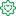# QlikView App Dev

Discussion Board for collaboration related to QlikView App Development.

Announcements
Skip the ticket, Chat with Qlik Support instead for instant assistance.
cancel
Showing results for
Did you mean:Specialist

## sum({1}sales) vs Total(Sum(sales))

Hi all,

Can u please tell me what is difference between sum({1}sales) vs Total(Sum(sales)).

Thanks

1 Solution

Accepted SolutionsMVP

Explanation given by Rob is fulfilling your requirements.

Let me give you a practical example.

Consider below script..

====================

Sales:

[

Customer, Sales, Brand

A, 100, B1

B, 120, B2

C, 90, B1

D, 110, B2

];

====================

Use Text objects to understand the logic...

=SUM(Sales)

The above expression gives you 420 but it will change according to your selection on Customer or Brand.

=SUM({1}Sales)

The above expression gives you 420 but it will not change according to your selection on Customer or Brand.

So the answer would be 420 even after selecting any dimension

=SUM(Total Sales)

The above expression will give you Total Sales ignoring dimension but if you select any dimension, it will change accordingly. SUM(Total Sales) is useful if you want to show Total Sales against each line in Pivot or Straight Table or in any other objects.

Create a Pivot Table

Dimensions

Customer

Brand

Expressions

SUM(Total Sales)

SUM(Total <Brand> Sales)

Here second expression will give you Total Sales Brand wise....

=SUM(All Sales)

Same as SUM({1}Sales)......

9 RepliesMVP

I think you meant

sum(TOTAL sales)

"TOTAL" means ignore dimension. For example, in a chart with Customer as the dimension, sum(TOTAL sales) would return the same number on every row. This can be useful for computing something like Customer pct of total as:

sum(sales) / sum(TOTAL sales)

{1} means ignore selections. For example, sum({1} sales) would return the same value regardless of selections made in Customer, Product or any other dimension. This is useful for computing pct of all sales like:

sum(sales) / sum({1}sales)

Lastly, the two can be combined to compute a row pct of all sales for the application, regardless of selection.

sum(sales) / sum{1} TOTAL sales)

-RobPartner - Specialist

The difference is that sum({1} sales) will not consider any selection you have in your app. It will always calculate the total amount of sales in your entire data, while the second, Sum(Total sales) (this is the right syntax) will calculate the total amount on the selected data.

EduardoMVP

Explanation given by Rob is fulfilling your requirements.

Let me give you a practical example.

Consider below script..

====================

Sales:

[

Customer, Sales, Brand

A, 100, B1

B, 120, B2

C, 90, B1

D, 110, B2

];

====================

Use Text objects to understand the logic...

=SUM(Sales)

The above expression gives you 420 but it will change according to your selection on Customer or Brand.

=SUM({1}Sales)

The above expression gives you 420 but it will not change according to your selection on Customer or Brand.

So the answer would be 420 even after selecting any dimension

=SUM(Total Sales)

The above expression will give you Total Sales ignoring dimension but if you select any dimension, it will change accordingly. SUM(Total Sales) is useful if you want to show Total Sales against each line in Pivot or Straight Table or in any other objects.

Create a Pivot Table

Dimensions

Customer

Brand

Expressions

SUM(Total Sales)

SUM(Total <Brand> Sales)

Here second expression will give you Total Sales Brand wise....

=SUM(All Sales)

Same as SUM({1}Sales)......Former Employee

The difference between {1} and total is explained in this blog post:

http://community.qlik.com/blogs/qlikviewdesignblog/2013/09/09/aggregation-scope

HICContributor III

Hello Manish,  I have same issue with a slightly different request.

i now have 2 dims order number and item number, but the point is i want to show the Sum(sales) for each item number but regardless the order number

To elaborate- I want to see the sum(sales) whenever i select an order number all the lines for that order come up but they show only the data for that order.

But what i want to do is show the Sum(sales) for all the items in that order regardless of the order number, Whatever is the entire sales for that item not only for that particular order.

But the users want to still filter it based on the order number and look what are the entire sales for all the items in that order. How can i achieve this.Partner - Creator

Hi, is there anyway to get sth. like that; sum({1} TOTAL <Dim1> sales), which i need is ignoring all dimensions except Dimension1 and ignore all selections. Syntax works but it doesn't calculate properly, i mean calculation is changing up to my selection. sum({1}TOTAL  <Dim1>sales) is not true or is there any way to get my answer.Partner - Creator

Hi, is there anyway to get sth. like that; sum({1} TOTAL <Dim1> sales), which i need is ignoring all dimensions except Dimension1 and ignore all selections. Syntax works but it doesn't calculate properly, i mean calculation is changing up to my selection. sum({1}TOTAL  <Dim1>sales) is not true or is there any way to get my answer.MVP

Berk, That should work as you described. Can you post a sample qvw that shows that shows the problem and the expected result?

-Rob